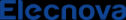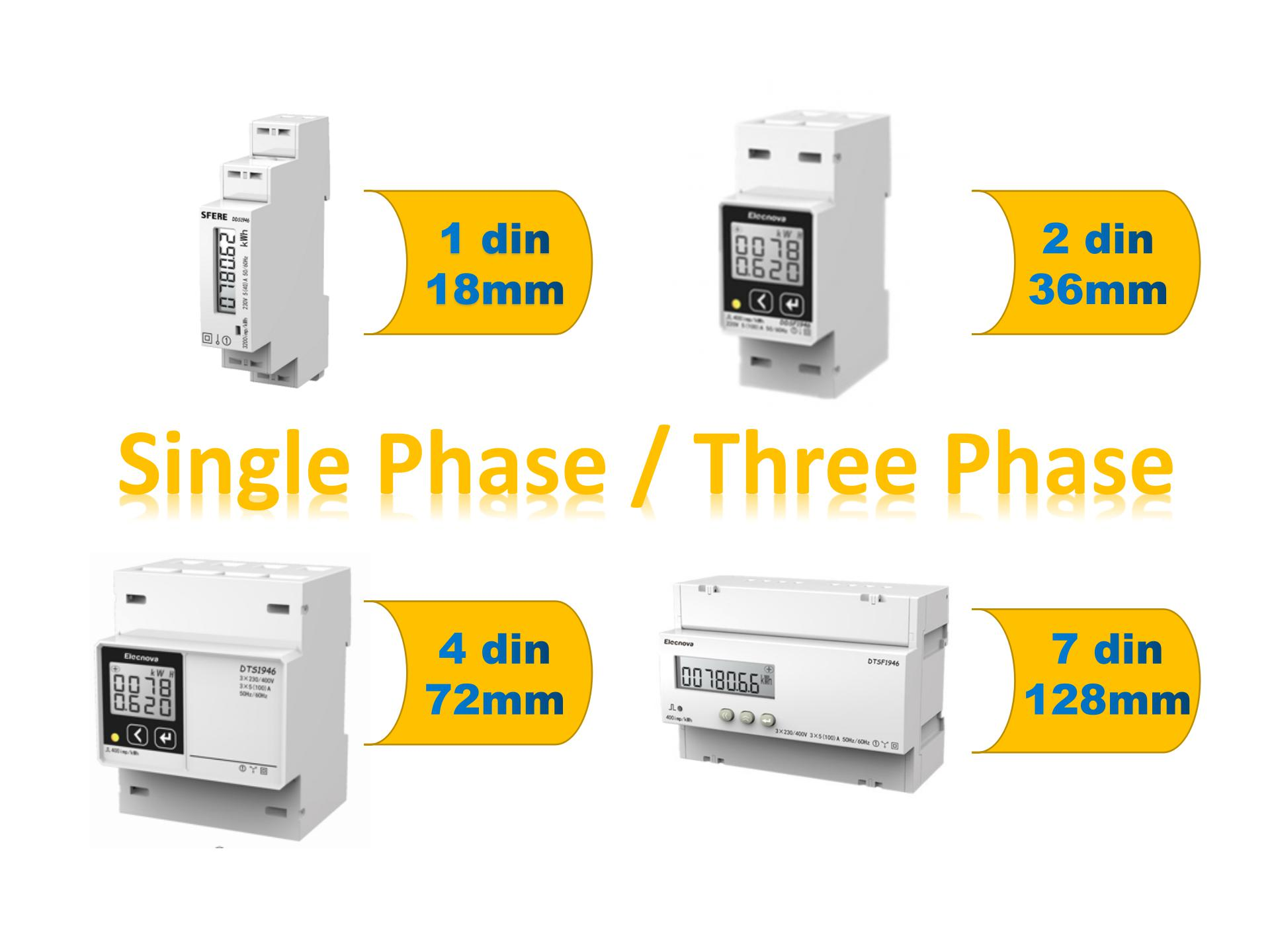# Digital power meter---A new trend for the residential power measuring

Electric energy meter is used to measure electric energy meter, also known as Kwh meter, refers to the instrument for measuring various electrical quantities.

1. What is electricity power meter

Electric energy meter is used to measure electric energy meter, also known as Kwh meter, refers to the instrument for measuring various electrical quantities. When using the electric energy meter, it should be noted that under the condition of low voltage (no more than 500 V) and small current (dozens of a), the electric energy meter can be directly connected to the circuit for measurement. In the case of high voltage or high current, the Kwh can not be directly connected to the line, but should be used with voltage transformer or current transformer

2. Categories of electricity power meters1) Digital power meter can be divided into DC current meter and AC current meter according to its circuit. AC power meter can be divided into single-phase electric power meter, 3P3W and 3P4W electricity power meter according to its phase and line connection.

2) According to its working principle, electricity can be divided into electrical mechanical electric energy meter and digital power meter. Electrical mechanical kwh meter is used in AC circuit. As an ordinary electric energy measuring instrument, the most commonly used one is induction type watt hour meter. Digital power meter can be divided into electronic kwh meter and electro mechanical kwh meter.

3) According to its structure, digital power meter can be divided into integral kwh meter and split kwh meter.

4) In terms of their uses, digital power meters can be divided into active power meter, reactive power meter, maximum demand meter, standard kwh meter, multi tariff digital power meter, prepaid digital power meter, and multi-functional digital power meter.

5) In terms of the measuring accuracy, the digital power meters can be divided into ordinary installation type (class 0.2, 0.5, 1.0, 2.0, 3.0 etc ) and portable precision level (Class 0.01, 0.02, 0.05, 0.1, 0.2 etc)

3. How power meter works?

When the electric energy meter is connected to the circuit under test, alternating current flows through the current coil and voltage coil. These two alternating currents generate alternating magnetic flux in their iron core respectively. The alternating magnetic flux passes through the aluminum plate and induces eddy current in the aluminum disc. The eddy current is acted by force in the magnetic field, so that the aluminum plate can get the torque (active torque) and rotate. The greater the power consumed by the load, the greater the current passing through the current coil, the greater the eddy current induced by the aluminum disc, and the greater the torque of the aluminum disc. That is, the torque is proportional to the power consumed by the load. The greater the power, the greater the torque, and the faster the aluminum disc rotates. When the aluminum disc rotates, it is also affected by the braking torque generated by the permanent magnet, which is opposite to the active torque; the brake torque is directly proportional to the rotational speed of the aluminum disc, and the faster the aluminum disc rotates, the greater the braking torque. When the active torque and braking torque reach a temporary balance, the aluminum disc will rotate at a constant speed. The power consumed by the load is proportional to the number of revolutions of the aluminum disc. When the aluminum plate rotates, it drives the counter to indicate the consumed electric energy. This is the simple process of electricity meters.

4. Advantages of digital power meter

The digital power meter use high integrated electronic integrated circuit, so it has great advantages in performance and operation function compared with induction meter.

1) Power consumption. Because the digital power meter is the combination of the electronic components , the power consumption of each meter is only about 0.6 ~ 0.7W. For the multi-user centralized digital power meter, the average power to each domestic household is smaller. Generally, the power consumption of each induction meter is about 1.7w

2) Accuracy. As far as the error range of the meter is concerned, the measurement error of class 2.0 digital power meter is ± 2% in the range of 5% to 400% of the calibrated current, and the accuracy level of 1.0 is widely used, and the error is smaller. The error range of induction watt hour meter is 0.86% ～ 5.7%. Moreover, due to the insurmountable defect of mechanical wear, the induction power meter goes slower and slower, and the final error is larger and larger. The State Grid of China has carried out spot checks on induction meters, and the results show that more than 50% of induction meters have exceeded the allowable range after being used for five years.

3) Overload, power frequency range. The overload of the digital power meter can reach 6 ~ 8 times, and has a wide range of measuring. At present, more and more users choose 8-10 times meter, some of which can even reach 20 times wide range measuring. The operating frequency is also wide, ranging from 40 Hz to 1000 Hz. However, the overload multiple of induction meter is only 4 times, and the working frequency range is only 45-55Hz.

4) Functionality. Because application of electronic technology, the digital power meter can be connected with the computer through the relevant communication protocols such as Modbus-RTU, Modbus-TCP, Profitbus-DP and BACnet etc. Meanwhile the hardware can be controlled and managed by programming software. Therefore, the smart meter not only has the advantages of compact size, but also has the functions of remote control, multiple tariff, identification of malignant load, anti stealing, prepaid electricity and other functions, and it can meet the different requirements of control functions by modifying different parameters in the control software, which are difficult or impossible for traditional induction meters

5. Summery

In general, digital power meter will gradually replace the traditional pointer meters and become the mainstream products of modern industrial measurement and control and civil electricity measurement with its advantages of high precision and fast data transmission. With the further widespread use of electronic information technology, especially the popularity of 4G, 5g mobile technology, the advantages of smart meters will be further reflected.